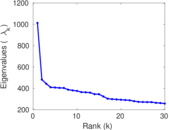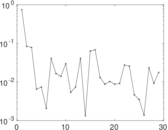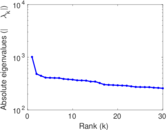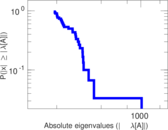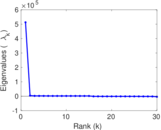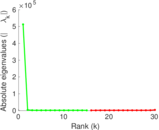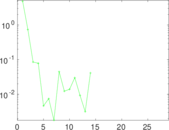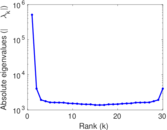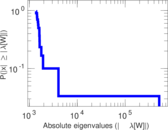# vi.sualize.us user–item

This is the bipartite picture tagging network of vi.sualize.us. Left nodes represent users and right nodes represents pictures. An edge connects a user with a picture he has tagged.

 Code `Vui` Internal name `pics_ui` Name vi.sualize.us user–item Data source http://vi.sualize.us/ AvailabilityDataset is available for download Consistency checkDataset passed all tests Category Interaction network Node meaning User, picture Edge meaning Tag assignment Network formatBipartite, undirected Edge typeUnweighted, multiple edges

## Statistics

 Size n = 512,524 Left size n1 = 17,122 Right size n2 = 495,402 Volume m = 2,298,816 Unique edge count m̿ = 997,840 Wedge count s = 489,164,625 Square count q = 19,336,325 4-Tour count T4 = 2,113,369,668 Maximum degree dmax = 46,571 Maximum left degree d1max = 46,571 Maximum right degree d2max = 790 Average degree d = 8.970 57 Average left degree d1 = 134.261 Average right degree d2 = 4.640 30 Average edge multiplicity m̃ = 2.303 79 Size of LCC N = 506,648 Diameter δ = 14 50-Percentile effective diameter δ0.5 = 5.057 31 90-Percentile effective diameter δ0.9 = 5.878 07 Median distance δM = 6 Mean distance δm = 5.154 40 Gini coefficient G = 0.776 842 Balanced inequality ratio P = 0.191 994 Left balanced inequality ratio P1 = 0.148 075 Right balanced inequality ratio P2 = 0.282 309 Relative edge distribution entropy Her = 0.838 461 Tail power law exponent γt = 2.201 00 Degree assortativity ρ = −0.074 900 8 Degree assortativity p-value pρ = 0.000 00 Spectral norm α = 1,012.77 Algebraic connectivity a = 0.004 221 18 Spectral separation |λ1[A] / λ2[A]| = 2.101 98 Controllability C = 478,505 Relative controllability Cr = 0.933 625

## Plots

### Fruchterman–Reingold graph drawing### Degree distribution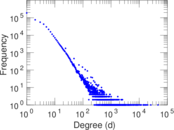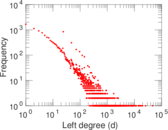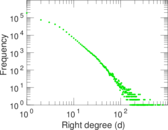### Cumulative degree distribution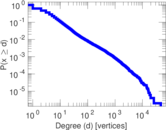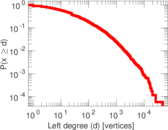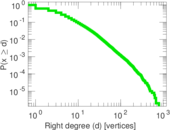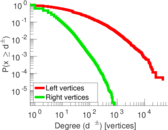### Lorenz curve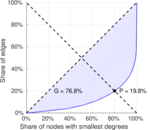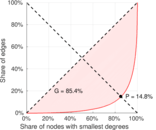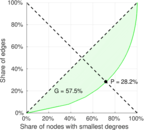### Spectral distribution of the adjacency matrix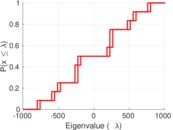### Spectral distribution of the normalized adjacency matrix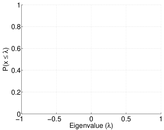### Spectral distribution of the Laplacian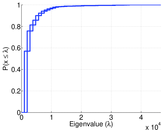### Spectral graph drawing based on the adjacency matrix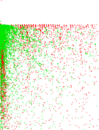### Spectral graph drawing based on the Laplacian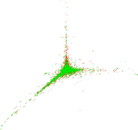### Spectral graph drawing based on the normalized adjacency matrix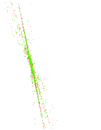### Degree assortativity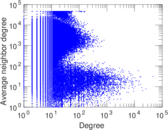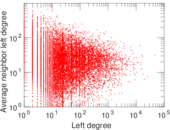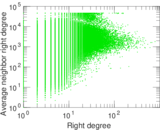### Zipf plot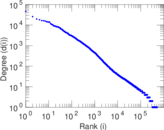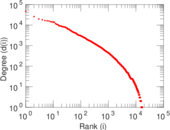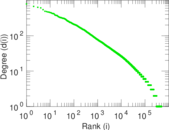### Hop distribution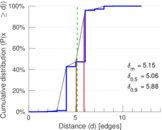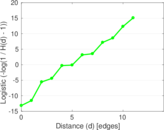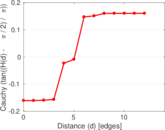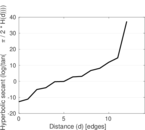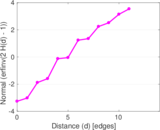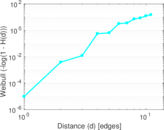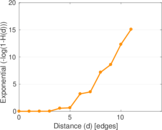### Delaunay graph drawing### Edge weight/multiplicity distribution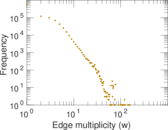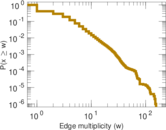### Matrix decompositions plots